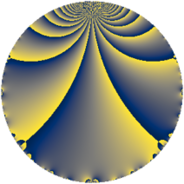# Properties

 Label 28.2.fLevel $28$ Weight $2$ Character orbit 28.f Rep. character $\chi_{28}(3,\cdot)$ Character field $\Q(\zeta_{6})$ Dimension $4$ Newform subspaces $1$ Sturm bound $8$ Trace bound $0$

# Related objects

## Defining parameters

 Level: $$N$$ $$=$$ $$28 = 2^{2} \cdot 7$$ Weight: $$k$$ $$=$$ $$2$$ Character orbit: $$[\chi]$$ $$=$$ 28.f (of order $$6$$ and degree $$2$$) Character conductor: $$\operatorname{cond}(\chi)$$ $$=$$ $$28$$ Character field: $$\Q(\zeta_{6})$$ Newform subspaces: $$1$$ Sturm bound: $$8$$ Trace bound: $$0$$

## Dimensions

The following table gives the dimensions of various subspaces of $$M_{2}(28, [\chi])$$.

Total New Old
Modular forms 12 12 0
Cusp forms 4 4 0
Eisenstein series 8 8 0

## Trace form

 $$4 q - 2 q^{2} - 6 q^{5} - 8 q^{8} + O(q^{10})$$ $$4 q - 2 q^{2} - 6 q^{5} - 8 q^{8} + 6 q^{10} + 12 q^{12} + 2 q^{14} + 8 q^{16} - 6 q^{17} + 6 q^{21} - 4 q^{22} - 12 q^{24} - 4 q^{25} - 12 q^{26} - 20 q^{28} + 16 q^{29} - 6 q^{30} + 8 q^{32} + 6 q^{33} - 6 q^{37} + 18 q^{38} + 12 q^{40} + 12 q^{42} + 4 q^{44} - 2 q^{46} - 4 q^{49} + 8 q^{50} + 2 q^{53} - 18 q^{54} + 16 q^{56} - 36 q^{57} - 8 q^{58} - 12 q^{60} - 18 q^{61} + 12 q^{65} - 6 q^{66} + 6 q^{70} + 30 q^{73} - 6 q^{74} - 10 q^{77} + 24 q^{78} - 24 q^{80} + 18 q^{81} + 12 q^{82} + 12 q^{85} - 4 q^{86} + 4 q^{88} + 54 q^{89} + 8 q^{92} + 6 q^{93} - 30 q^{94} - 24 q^{96} - 22 q^{98} + O(q^{100})$$

## Decomposition of $$S_{2}^{\mathrm{new}}(28, [\chi])$$ into newform subspaces

Label Dim $A$ Field CM Traces $q$-expansion
$a_{2}$ $a_{3}$ $a_{5}$ $a_{7}$
28.2.f.a $4$ $0.224$ $$\Q(\zeta_{12})$$ None $$-2$$ $$0$$ $$-6$$ $$0$$ $$q+(-\zeta_{12}-\zeta_{12}^{2}+\zeta_{12}^{3})q^{2}+(\zeta_{12}+\cdots)q^{3}+\cdots$$